and#8216;Redshiftand#8217; in Electromagnetic Waves | OMICS International
Fluid Mechanics: Open Access

# ‘Redshift’ in Electromagnetic Waves

David John Smith1*

1Retired Engineer, Rotherham, United Kingdom.
BSc and ARCS were awarded in 1973 by the University of London, Imperial College, United Kingdom.

*Corresponding Author:
Smith DJ
Retired Engineer
Rotherham
United Kingdom
Tel: +44 7966547679
E-mail: [email protected]

Received Date: August 11, 2016; Accepted Date: August 20, 2016; Published Date: August 28, 2016

Citation: Smith DJ (2016) ‘Redshift’ in Electromagnetic Waves. Fluid Mech Open Acc 3:131.

Copyright: © 2016 Smith DJ. This is an open-access article distributed under the terms of the Creative Commons Attribution License, which permits unrestricted use, distribution, and reproduction in any medium, provided the original author and source are credited.

Visit for more related articles at Fluid Mechanics: Open Access

#### Abstract

Electromagnetic waves are commonly described in terms of Maxwell’s Equations but it is often neglected that an essential part of Maxwell’s theory was that such waves propagate through an ethereal medium. According to Maxwell, it is the electromagnetic properties of this medium that determine the speed of light. As the aether has never been detected it has been conveniently ignored for over a century. However, since the 1990’s it has been accepted that around 70% of the mass energy density of the universe can be attributed to “dark energy”. This article considers that aether and dark energy are alternative descriptions of the same entity. By considering the propagation of spherical waves through such a medium, it is shown that the aether should have a finite electrical conductivity as well as the magnetic permeability and electrical permittivity considered by Maxwell. This finite conductivity results in thermal dissipation and a loss of transmitted energy giving a consequential ‘redshift’ in frequency and wavelength of electromagnetic waves. This ‘redshift’ is compared to that detected in astronomical observations of distant galaxies. It is concluded that this could provide an alternative explanation for phenomena that have been used to justify the current model of the universe.

#### Keywords

Aether; Dark energy; Electromagnetic wave; Maxwell’s equations; Redshift

#### Introduction

Electromagnetic waves are commonly described in terms of Maxwell’s Equations, named after James Clerk Maxwell, who presented his “Dynamical Theory of the Electromagnetic Field” to the Royal Society in December 1864 .

An essential part of Maxwell’s theory was that:

“There is an ethereal medium filling space and permeating bodies, capable of being set in motion and of transmitting that motion from one part to another, and of communicating that motion to gross matter so as to heat it and affect it in various ways.”

Maxwell’s theory was championed by Oliver Heaviside, who further developed it in his “Electromagnetic Theory” published in three volumes in 1893, 1899 and 1912 respectively [2-4]. Heaviside used a method of vector analysis to simplify Maxwell’s original equations and reiterated the essential function of the aether.

Since the 1990’s it has generally been accepted that around 70% of the mass energy density of the universe can be attributed to “dark energy”. This dark energy is described as a cosmological constant (Lambda) in the Standard Cosmological Model or as a dynamic entity (Quintessence – fifth element) in alternative models. I would suggest that Aether, Ether, Lambda, Quintessence and Dark Energy are all alternative descriptions of the same entity.

#### Maxwell’s Equations

Heaviside’s use of vector algebra and linear operators for describing electromagnetic theory in terms of electric and magnetic field vectors led to the description of Maxwell’s equations recognized today. This essentially reduces to four equations that can be expressed as: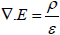(1)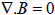(2)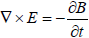(3)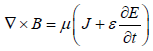(4)

Where, in SI units, ∇ is the vector differential operator per metre (m-1), E is the electric field vector in Volts per metre (Vm-1), ρ is the charge density in Coulombs (Amps seconds) per cubic metre (Asm-3) and ε is the permittivity of the medium in Coulombs per Volt per metre (AsV-1m-1). B is the magnetic field, a pseudo vector field in Volts seconds per square metre (Vsm-2). J is the current density in Amps per square metre (Am-2) and μ is the magnetic permeability of the medium in Volts seconds per Amp per metre (VsA-1m-1).

If we also consider Ohm’s Law:

J=σE     (5)

Where σ is the conductivity of the medium in Amps per Volt per metre (AV-1m-1).

Equations (4) and (5) then give: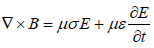(6)

The wave equation for a conducting medium is then: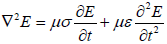(7)

If the medium is non-conducting, with σ=0, this becomes the standard wave equation: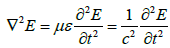(8)

Where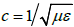is the speed of propagation.

A general plane wave sinusoidal solution of this equation is given as: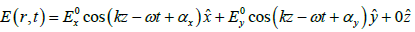(9)

Where r = (x, y, z) is a position vector, the wave is propagating in the z direction,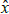,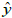and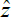are unit vectors in the x, y and z directions respectively,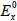and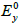are initial amplitudes, k is the wave number, ω = ck is the angular frequency, c is the speed of propagation, t is the time variable and αx and αy are initial phases.

#### Spherical Electromagnetic Waves

Consider a spherical region of space containing a number of sources of electromagnetic waves. The sphere could be any size and the sources could be anything from individual photons to complete stars or galaxies. The only condition is that the sources are completely enclosed by the sphere and the centre of the sphere is the effective centre of the combined sources (Figure 1).

According to Maxwell’s theory (described in terms of vectors), every electromagnetic wave from every individual source within this sphere can be represented by an electric field vector, with an associated magnetic field that is perpendicular to and proportional to the electric field. Both fields are mutually perpendicular to the direction of wave propagation.

An important point to note about vectors is the result of addition. The same result can be obtained by the addition of any number of different component vectors. Other important considerations are concepts of zero and infinity. Any value that is less than the resolution of a measuring system can be considered to be zero. Similarly, infinity can be considered as any value greater than the maximum value that can be handled by the measuring system. Zero and infinity are inversely proportional to each other and can be considered to be parameters determined by the measuring system. Heaviside pointed out that by using his methods for manipulating vectors and linear operators, individual components, when considered in isolation, could have zero or infinite values yet the final result could still be finite.

This means that it is not necessary to know microscopic details of all individual component vectors if it is the final sum that is of interest. Such vectors may be discontinuous and may be determined to have zero or infinite values if considered in isolation. Likewise, it is not necessary to know microscopic details of properties of the medium. The medium could be discontinuous with apparently infinite changes in microscopic properties. If such changes are at a scale that is outwith the scope of the measuring system, then measured quantities are an average of all the individual components.

At any point within the sphere, individual vectors with a common frequency, from all sources, can be combined by vector addition. At the surface of the sphere the net result, for each common frequency, is a combined wave propagating normal to the surface away from the centre of the sphere. The electric and magnetic field vectors are directed in a plane which is tangential to the surface of the sphere.

If the number and distribution of sources within the sphere is spherically symmetrical, any point on the surface of the sphere is equivalent to any other point on the surface. Any direction of orientation of the sphere is equivalent to any other direction of orientation. In view of this symmetry, consider a circularly polarized simple harmonic wave. Taking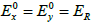and αx = 0, αy = π/2, a circularly polarized wave at the surface of the sphere can be expressed in spherical coordinates as: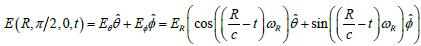(10)

Where E(R,π 2,0,t) is the electric field vector tangential to the surface of the sphere and R is the radius of the sphere. The chosen point is set to be on the effective equator of the sphere, with a polar angle of θ =π/2 , and an azimuthal angle, Ï�, of zero. The time variable is t, Eθ and Eφ are directional components of the electric field vector and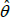and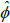are unit vectors in the associated directions. ER is the amplitude of the electric field, c is the speed of propagation and ωR is the angular frequency.

The magnetic field is proportional to and is perpendicular to the electric field and is also tangential to the surface of the sphere. The field directions rotate at the angular speed ωR (Figure 2).

The linear frequency of the wave is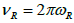and the wavelength is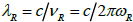.

At any other point on the surface of the sphere the field vectors will have the same amplitude but will have a phase difference relative to that at the point θ = π/2, Ï�=0 (Figure 3).

The electric field vector, with a phase difference of φ, is: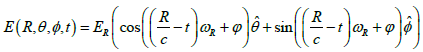(11)

There will be an equal and opposite field vector at a distance of half a wavelength in any direction giving transverse waves with the same frequency and wavelength as the forward wave.

Using the spherical law of cosines we can take the phase difference to be: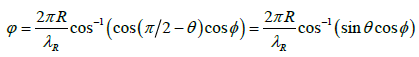(12)

The field vectors will thus form a regular pattern over the surface of the sphere (Figure 4).

As the field vectors rotate, this pattern will rotate (Figure 5).

The electric field vector gives rise to an electric current vector with two components: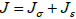(13)

The first component, Jσ = σE , is in phase with the electric field. It is due to the conductivity of the medium (σ) and follows the transverse waves. The second component, Js = ε∂E/∂t , is proportional to the rate of change of the electric field. This is the capacitive component due to the permittivity of the medium (ε).

The power in the wave will similarly have two components. The power density due to the conduction current will be the scalar product of the electric field and conduction current vectors: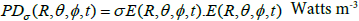(14)

The scalar product of two vectors is the sum of the products of the directional components. Therefore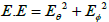and, using the identity sin2+ cos2 = 1, this gives a power density due to the conductivity of the medium: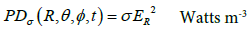(15)

This is real power and is dissipated as heat.

The capacitive current vector is: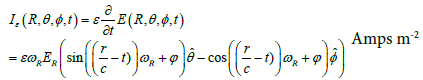(16)

The electric field and capacitive current vectors are out of phase and the scalar product is zero. In this case the power is reactive and it is defined as the product of rms voltage and rms current. This gives a reactive power density of: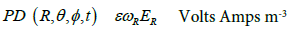(17)

The total power density in the wave at the surface of the sphere is: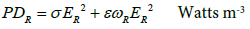(18)

This power is contained in a spherical shell with an outer radius, R, and an inner radius, R −λR (Figure 6).

The volume of this shell is: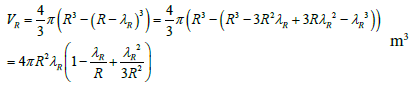(19)

Therefore the total power in the wave at radius R is: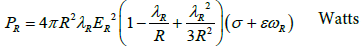(20)

The power due to the conduction current is dissipated to the medium and the reactive power is transmitted forward to a shell with inner radius R, and an outer radius, R + λR.

The total power density in the wave at r = R + λR is: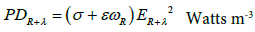(21)

The volume of this shell is: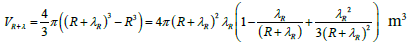(22)

Therefore the total power in the wave at radius R + λR is: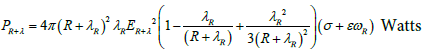(23)

The reactive power at radius R becomes the total power at R + λR and therefore: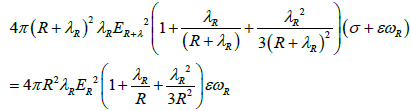(24)

For R>>λ, terms with λR/R and λR2/R2 can be neglected and dividing both sides by 4π gives: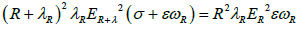(25)

Rearranging gives: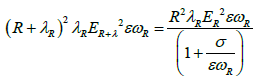(26)

Using λω = c / 2π and considering δr = λR as a unit distance increment, this can be written as: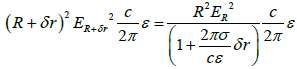(27)

Let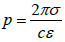and divide both sides by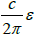to give: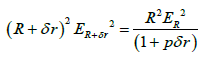(28)

Following the same process for r = R + 2δr gives: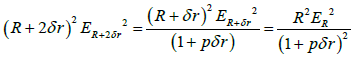(29)

Continuing to r = R + nδr gives: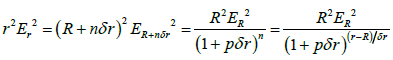(30)

Note that as δr is a distance increment, the increments are not necessarily complete wavelengths. However, this does not affect the proportion of dissipated power relative to transmitted power in each increment.

In terms of the rotating electric field vector, the loss of power due to conduction is analogous to the loss of power in a rotating mechanical system due to friction. With a spinning wheel, the speed reduces linearly with distance due to friction and likewise the speed of rotation of the electric field vector reduces linearly with distance.

Observations of waves from distant galaxies have shown that frequency and wavelength are shifted and that the ‘redshift’ is proportional to distance giving: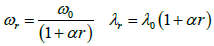(31)

Where α = 7.2751×10-27 m-1 is the Hubble constant, as determined by the Planck satellite mission  expressed in SI units, and r is distance in metres.

From the standard solution to the wave equation, a change in electric field vector is proportional to the square of a change in frequency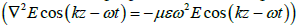and as power is proportional to the square of the electric field vector then a change in power is proportional to the fourth power of a change in frequency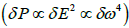.

Observations of transmitted power in terms of the surface brightness of distant galaxies [6,7] have shown a relationship between power in the wave and distance from the galaxy of the form: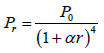(32)

Therefore, as transmitted power,Pr, is proportional to r2Er2 :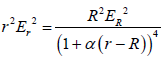(33)

Then comparing (30) with (33):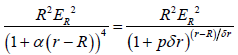(34)

Rearranging, taking the 4th root and using a series expansion gives: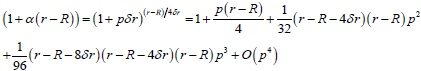(35)

If terms with p2 and higher powers can be neglected then: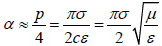(36)

Using c = 2.9979×108 msâ��1 and ε = 8.854187817 ×10-12 AVâ��1mâ��1 gives conductivity of the aether as: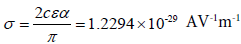(37)

The ratio of transmitted power compared to the power dissipated by conduction for a frequency of ν = 5.44×1014 s-1 (the midpoint of the visible band) becomes: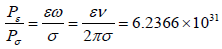(38)

#### Waves from Finite Sources

The above discussion assumes spherically symmetric waves but this need not be the case. It is often said that light travels in straight lines. I would prefer to say that light travels in spherical waves and that the normal to the wavefront is a straight line path unless the shape of the wavefront is distorted by reflection, refraction or diffraction. The parameter r used in the previous section is taken to be the distance from the source but if the shape of the wavefront is changed then r represents the curvature of the wavefront. Similarly, the assumption of circular polarization is a natural form consistent with photon spin and this simplifies the description but it is not necessary. The same result would apply for any wave of any frequency with any form of polarization. The assumption of symmetry merely serves to simplify calculations. There is always a loss of transmitted power due to the conductivity of the medium irrespective of the shape of the wavefront.

The cross section area of the wavefront that is actually detected by an observer is only a small fraction of a spherical surface. The remainder of the wavefront is of no consequence unless the wave is redirected towards the observer. An actual source could be any shape. The spherical shape of the wavefront is not necessarily complete if the wave is obstructed in another direction.

For a finite object, each resolvable point on the surface of the object can be considered as a source of spherical waves. These waves may be emitted by the object or they may be a reflection of incident waves. In the case of reflected waves, the shape of the wavefront and the power distribution across it, depends on the shape and colour of the reflecting surface as well as properties of the incident waves.

The parameter R used in the previous section is the radius of an arbitrarily defined imaginary sphere and in reality it is determined by the resolution of the detector. For example, if an image of an object is collected on a digital camera, then each pixel of the image represents a point on the object and so R is determined by the pixel size of the image.

If waves are refracted by a convex lens or reflected from a concave surface then expanding spherical waves can be changed to contracting spherical waves when the waves are directed towards a focus. If the waves then continue past the focal point they become expanding waves again.

In the case of diffraction through a pinhole, the pinhole becomes a source of spherical waves but with a strong energy bias in the original direction.

#### Conclusions

The existence of an ethereal medium is fundamental to Maxwell’s theory and it is the permittivity (ε) and permeability (μ) of this medium that determine the speed of light. By considering the propagation of spherical waves we find that a finite conductivity (σ) is also necessary. However, the aether has been conveniently ignored for many years and the effect of conductivity is not generally significant unless propagation over distances measured in intergalactic proportions is considered.

It is only relatively recently that the existence of dark energy has been acknowledged to be necessary for models of the universe to be developed. If the universe is filled with dark energy that behaves as an ethereal medium, with uniform electromagnetic properties determined as conductivity (σ), permittivity (ε) and permeability (μ), and these properties can be considered to be constant values irrespective of any linear motion through the medium, then:

Electromagnetic energy propagates in spherical waves.

The speed of propagation is constant and is determined as: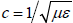.

As waves propagate, energy is consumed as a consequence of the electrical conductivity of dark energy. Electromagnetic energy is dissipated to dark energy as heat. The energy propagated is reduced by the amount dissipated. The rate of reduction approximates to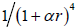. Where r is the radius of curvature of the wavefront and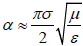.

The wave frequency (ν = 2πω ) reduces at a rate that approximates to 1 (1+αr ) .

The wavelength (λ = c/ν ) increases at a rate that approximates to (1+αr ) .

Time intervals, measured as the inverse of frequency (τ =1/ν ) , increase at a rate that approximates to (1+αr ).

Microscopic variations in properties of the ethereal medium are of no consequence to finite wave properties.

In my book “Where There’s Dark…” , I consider ‘redshift’ of spherical waves in terms of forces between peaks of electromagnetic energy. This is entirely consistent with an analysis in terms of field vectors as any two mathematical approaches that give the same result from the same initial conditions can be shown to be equivalent. In the book I consider the implications of such a ‘redshift’ and conclude that all the phenomena that have been used to justify an expanding universe theory based on an initial ‘Big Bang’ can have alternative explanations based on spherical waves propagating through a medium of dark energy.

#### Acknowledgements

Information used for preparation of this article is freely available via the internet and in particular www.wikipedia.org. I would like to thank all the individual contributors for sharing their knowledge.

#### References

Select your language of interest to view the total content in your interested language

### Article Usage

• Total views: 9633
• [From(publication date):
October-2016 - Mar 31, 2020]
• Breakdown by view type
• HTML page views : 9450Can't read the image? click here to refresh